## Bringing interactivity to network visualization in Jupyter notebooks: visJS2Jupyter

September 30, 2016

Brin Rosenthal (sbrosenthal at ucsd.edu)

### Introduction

Data is everywhere these days, and being able to interact with visual representations of that data in real time can help bring it to life.  You have to look no further than the D3 (data-driven-documents) examples page to see this.  If you haven’t spent time browsing through the D3 examples library, I would highly recommend doing so, but be warned it is easy to spend a few captivating hours here! (A few of my favorites: collision avoidancecollapsible force layout,  NCAA march madness predictionspreferential attachment).

Unfortunately, D3 is pretty nontrivial to learn, which can be a significant barrier to those of us looking for a quick but awesome solution.  There are some good visualization libraries which are based on D3, and simpler to use.  One of our favorites is vis.js.

If you’re anything like me, you love the fast and flexible development and documentation environment that Jupyter notebooks provide.  But I had been frustrated with the limited interactivity that is available for plotting of data.  While matplotlib, seaborn, and networkx provide nice static ways of graphing data and networks, they left me wanting more.  Python widgets are ok, but a bit clunky (see earlier post…) .

A group of us at the CCBB had the idea to write a tool which would bring the interactivity of D3 (through vis.js) into Jupyter notebook cells.  This turned out to be quite simple.  We repurposed some existing html code from another project, to set the styles of nodes and edges in a network.  We modified this code to allow style arguments to be passed in through a function.  Every time this function is called, a new style_file.html is created, containing the properties set by the user.  This style_file.html is then loaded into the Jupyter cell using the python HTML module, and the network is rendered in the cell.  Once we figured these pieces out, we had a fully interactive graph!  Right there in the Jupyter notebook cell!  We can now freely pan, zoom, click and drag nodes, and even embed more information in the node and edge hover-bubbles.  One of the coolest things about this tool is that it is almost infinitely flexible, and we’ve designed it to work with networkx graph formats- are one of the most standard python graph libraries.

In this post, I’ll walk you through two simple examples of how to use visJS2Jupyter.

### Installation

To install, run “pip install visJS2jupyter” in your terminal. To import, use the statement “import visJS2jupyter.visJS_module” in your notebook.  Source code for the package may be found here https://github.com/ucsd-ccbb/visJS_2_jupyter.

### Use example with default parameters

Now that we have the package installed, we’re going to walk through a very simple use example, using only the default parameters.  First, we need a network to draw.  Let’s make a random one using the networkx function ‘connected_watts_strogatz_graph’.  This network has 30 nodes, each of which is initially connected to 5 nearest neighbors.  Each of these connections randomly rewired with probability 0.2.  We will also need the lists of nodes and edges that comprise this graph.

``````
G=nx.connected_watts_strogatz_graph(30,5,.2)
nodes = G.nodes()
edges = G.edges()
``````

Next, we will simply construct dictionaries which contain all of the node-specific and edge-specific traits which will be passed to the visualizer.  (Note that we also need to make a node_map here, which maps the names of the nodes in the graph to integers, because of the way visJS interprets node/edge data).

``````    nodes_dict = [{"id":n} for n in nodes]
node_map = dict(zip(nodes,range(len(nodes)))) # map to indices for source/target in edges
edges_dict = [{"source":node_map[edges[i]], "target":node_map[edges[i]],
"title":'test'} for i in range(len(edges))]

``````

Now all that’s left is calling the visualizer function:

``````
visJS_module.visjs_network(nodes_dict, edges_dict, time_stamp=0)

``````

Done! Now we are free to click, drag, and zoom at will. Note that if you click on a node, that node’s nearest neighbors are highlighted.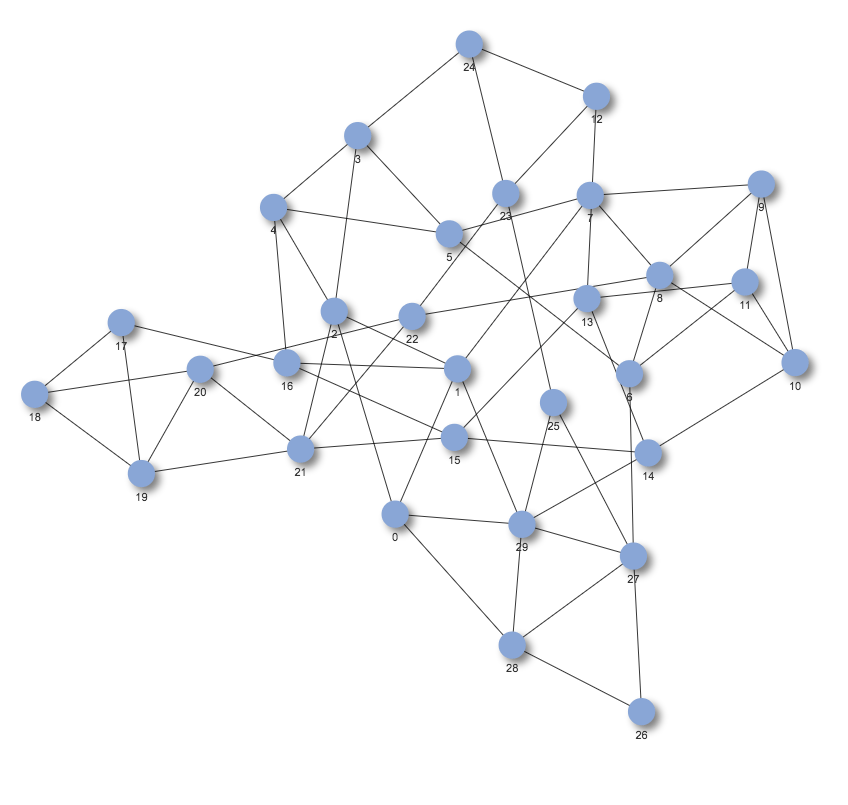Now that we have the basic use example under our belt, let’s move on to something more complicated, because there is so much potential here!

### More complicated use example

In this example, we will start by mapping some features to node and edge properties.  To map node/edge attributes to properties, simply add the property to the graph as a node/edge-attribute (using nx.set_node_attribute and nx.set_edge_attribute), then use the return_node_to_color function to select which property you would like to map to the node colors.  You can map anything you want to node color, as long as you represent it numerically.  You can also choose which matplotlib colormap  you’d like to use for the mapping.  For example, let’s calculate the node-level clustering coefficient and betweenness centrality and degree for our random network we made above, and add them as attributes.

``````
# add a node attributes to color-code by
cc = nx.clustering(G)
degree = G.degree()
bc = nx.betweenness_centrality(G)
nx.set_node_attributes(G,'clustering_coefficient',cc)
nx.set_node_attributes(G,'degree',degree)
nx.set_node_attributes(G,'betweenness_centrality',bc)
``````

Now that we’ve added each of these properties as node attributes, let’s map the node colors to betweenness centrality, and use the matplotlib colormap spring_r for our color scheme. We can also set the node transparency, using alpha, (1 = fully opaque, 0 = fully transparent), and we can choose which section of the colormap we’d like to use. Here we’re setting the lowest value of betweenness centrality to 10% of spring_r, and the highest value to 90%. This is useful if you like most of a colormap, but only want to use the part you like (if it starts too light or too dark for example). You can also transform your color scale, using the ‘color_vals_transform’ argument. Valid options are ‘log’, ‘sqrt’, and ‘ceil’.

``````
node_to_color =   visJS_module.return_node_to_color(G,field_to_map='betweenness_centrality',cmap=mpl.cm.spring_r,
alpha = 1, color_max_frac = .9,color_min_frac = .1)

``````

Now that we have our color mapping, we can fill out nodes_dict, node_map, and edges_dict, as we did in the simple example. This time, however, we will set more node and edge level properties, including:

• the positions of each node (x and y) using the output from nx.spring_layout
• The color of each node using our color mapping node_to_color
• The degree of each node (if degree is passed in, it is used to map node size by default)
• We’ll pass in dummy values for the node title field (this is what will show up in the hover).
• The color of each edge (for now we set every edge to be the same color- gray, but you can easily individualize the edge colors too, using visJS_module.return_edge_to_color(…)).

This is the current list of properties you can modify at the node level

• ‘node_shape’
• ‘color’
• ‘border_width’
• ‘title’ (e.g. the hover information)
• The default node size is mapped to the node degree, but you can override that default by setting ‘node_size_field’ in the visjs_network function.  For example, simply add a ‘node_size’ key:value entry to the nodes_dict, and call visjs_network with node_size_field = ‘node_size’.
• ‘degree’: the degree of each node- used for default size mapping
• All of the above are optional additions to nodes_dict.  Default values will be filled in if they are missing.

``````
pos = nx.spring_layout(G)
nodes_dict = [{"id":n,"color":node_to_color[n],
"degree":nx.degree(G,n),
"x":pos[n]*1000,
"y":pos[n]*1000} for n in nodes
]
node_map = dict(zip(nodes,range(len(nodes))))  # map to indices for source/target in edges
edges_dict = [{"source":node_map[edges[i]], "target":node_map[edges[i]],
"color":"gray","title":'test'} for i in range(len(edges))]

``````

We’ll also pass in some more graph-level properties (properties that aren’t node and edge specific). These include:

• node_size_multiplier: multiply each node’s size by this (useful if you have very few or very many nodes)
• node_color_highlight_border
• node_color_highlight_background
• node_color_hover_border
• node_color_hover_background
• node_font_size
• edge_arrow_to: Should we draw arrows at the target end?
• edge_color_highlight
• edge_color_hover
• edge_width: how wide should the edges be?
• physics_enabled, min_velocity, max_velocity: controls the physics of the nodes
• Time_stamp: This appends the value to the end of the style-file, thus creating a new one instead of writing over the old one.  You need a unique style-file for every network you render within the same Jupyter notebook.

We have mapped most (still working on getting the complete list) of the modifiable fields from visJS network into our package.  You can find documentation on the full list here .

``````
visJS_module.visjs_network(nodes_dict,edges_dict,time_stamp=1,
node_size_multiplier=5,
node_size_transform = '',
node_color_highlight_border='red',
node_color_highlight_background='#D3918B',
node_color_hover_border='blue',
node_font_size=25,
edge_arrow_to=True,
edge_color_highlight='#8A324E',
edge_width=3,
physics_enabled=True,
min_velocity=1,
max_velocity=15)
``````

Ok there we go! Now we have drawn a much more interesting network.  Click on the image below to be redirected to the interactive version, hosted on bl.ocks.org.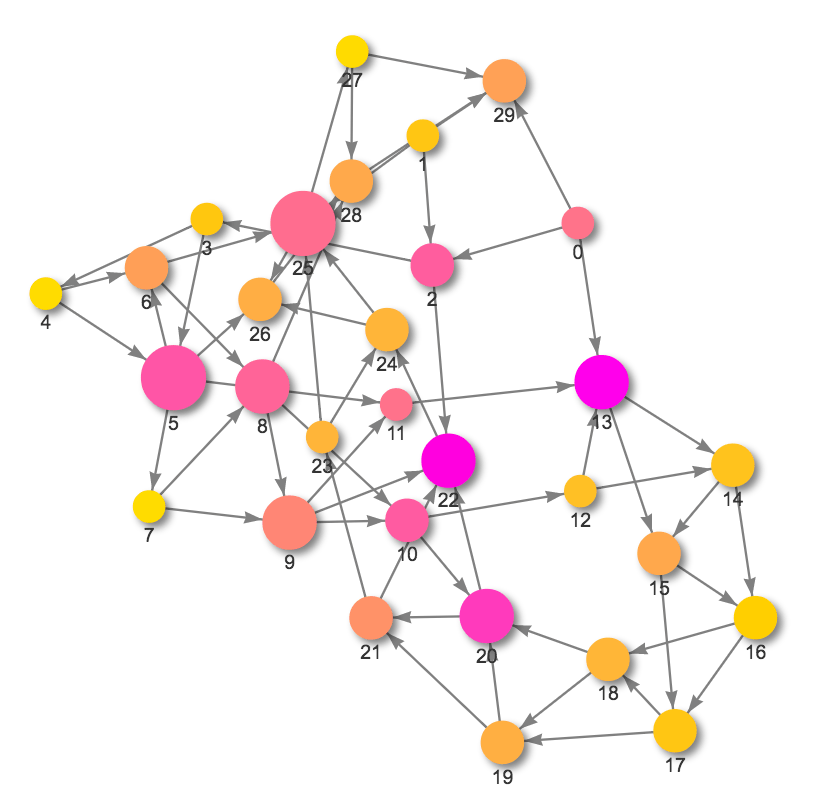For an even more complicated use case, see this notebook I wrote (http://bl.ocks.org/brinrosenthal/raw/fd7d7277ce74c2b762d3a4d66326215c/).  In this example, we display the bipartite network composed of diseases in The Cancer Genome Atlas (http://cancergenome.nih.gov/), and the top 25 most common mutations in each disease. We also overlay information about drugs which target those mutations. Genes which have a drug targeting them are displayed with a bold black outline. The user may hover over each gene to get a list of associated drugs.

## Outputting Beautiful Jupyter Notebooks (R-Kernel Edition)

September 13, 2016

Amanda Birmingham (abirmingham at ucsd.edu)

Jupyter notebooks are wonderful, but eventually you will need to present your work to someone unable (or unwilling) to view it on a notebook server. Unfortunately, there are surprising difficulties in printing or otherwise outputting Jupyter notebooks attractively into a static, offline format. These difficulties are not limited to Python-kernel notebooks: R-kernel notebooks have their own issues. Here’s a description of those issues, and a work-around that doesn’t require learning to modify jinja2 templates.

### HTML Output: Mangled Graphics Text

At first blush, it looks as though the HTML conversion built into Jupyter notebooks (shown below) works fine for R-kernel notebooks, as no errors are thrown and the output generally looks attractive.However, as you scroll through your document, you will find that something sinister has happened to plots after the first plot that uses legends/axis labels. For example, in my sample notebook, the first plot with a legend looks great:… but all subsequent ones have some of their text labels sadly mangled (e.g., look at the legend at the far right of the plot below):Apparently the cause of this mess is that (a) the Jupyter R kernel, `IRKernel`, by default outputs all graphics as inline SVG, but (b) the `nbconvert` tool that Jupyter uses to create HTML doesn’t "honor the ‘isolated’: true flag" in the metadata that tells it to put the SVG in its own iframe (about half of this statement is Greek to me, but feel free to get more details from the horse’s mouth–the `nbconvert` issue itself is at https://github.com/jupyter/nbconvert/issues/129, and is still open as of 08/25/2016).

### PDF Output: Line Truncation

So, let’s just output as PDF, which also "works" (i.e., doesn’t error out) for R-kernel notebooks, right?Wrong! In PDFs, it is true that the plots all look lovely: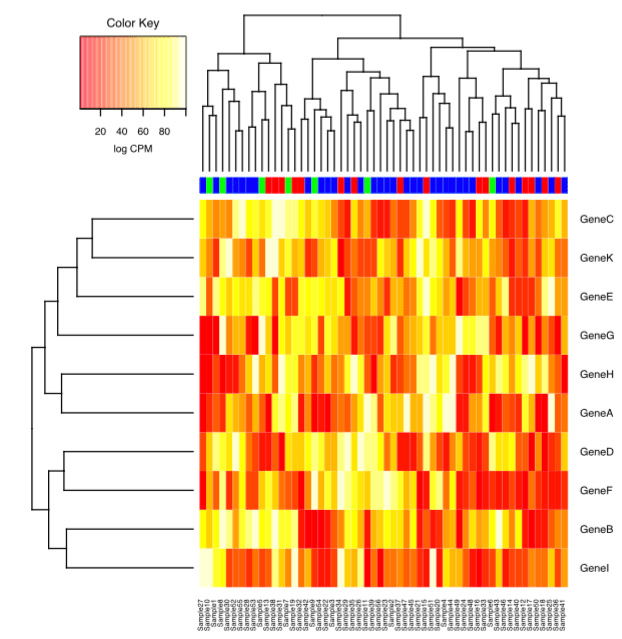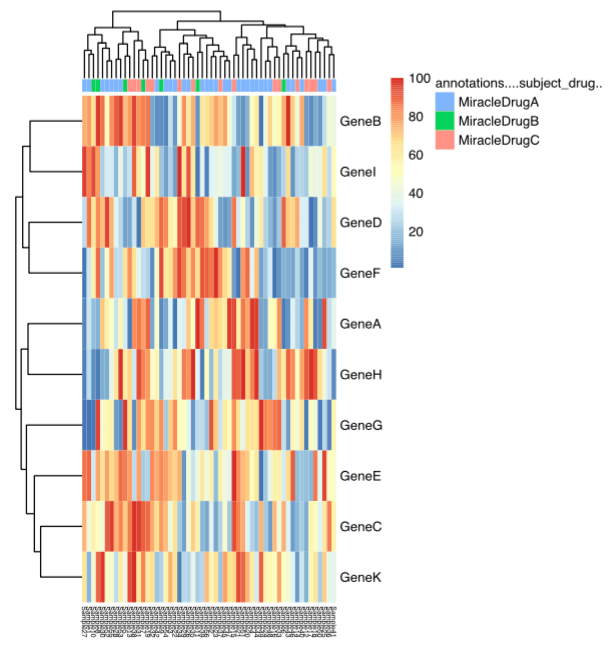However, something else has gone off the rails! The HTML output, for all its plot failures, does pretty well with text: it tries (and often succeeds in) coercing tables to fit the screen size, and when that fails adds a scrollbar to allow access to content too wide for the screen:Unfortunately, no such grace is forthcoming from the PDF output: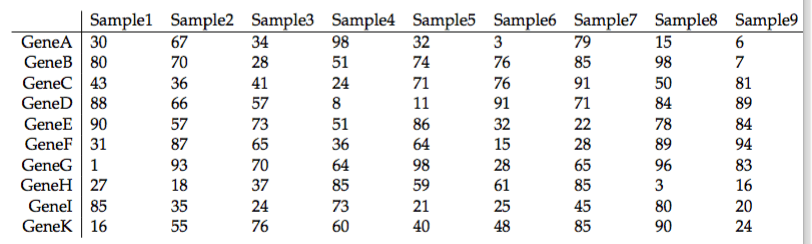As shown by the gray bar at the right of each of these screenshots, long content, whether tabular or textual, simply runs off the edge of the pdf page (a problem, sadly, that plagues all Jupyter notebooks regardless of kernel, as they all use `nbconvert` to make PDFs). Oh, the humanity!

### Workaround: HTML to PDF Without SVG

Fortunately, this mishigas can be side-stepped with minimal loss of quality and sanity. As described at https://github.com/IRkernel/IRkernel/issues/331, simply add this line to the top of your R-kernel notebook:

`options(jupyter.plot_mimetypes = c("text/plain", "image/png" ))`

Then be sure to restart your kernel so it takes effect:What effect? Well, it tells IRKernel to stop trying to output all graphics as SVG (by the way, this is also helpful if you have very LARGE plots that are bloating the on-disk size of your notebook or causing it to hang when rendered). You are instead telling it to make all graphics inline PNGs. PNGs look just slightly different than their SVG counterparts–a little blockier, since the former are raster graphics while the latter are vector graphics. I notice it most in the text:

SVGPNGBut what if you need a PDF? Well, with the HTML conversion licked, we can now get an acceptable PDF by simply opening the HTML version of the notebook in the browser and using the browser’s ability to "print" it to PDF (as shown here in Chrome):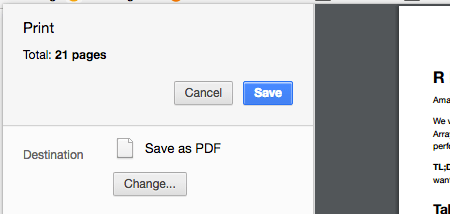This gives us unmangled plots (that, by the way, are appropriately placed so they aren’t broken across pages–unlike PDFs created from HTML by tools such as wkhtmltopdf):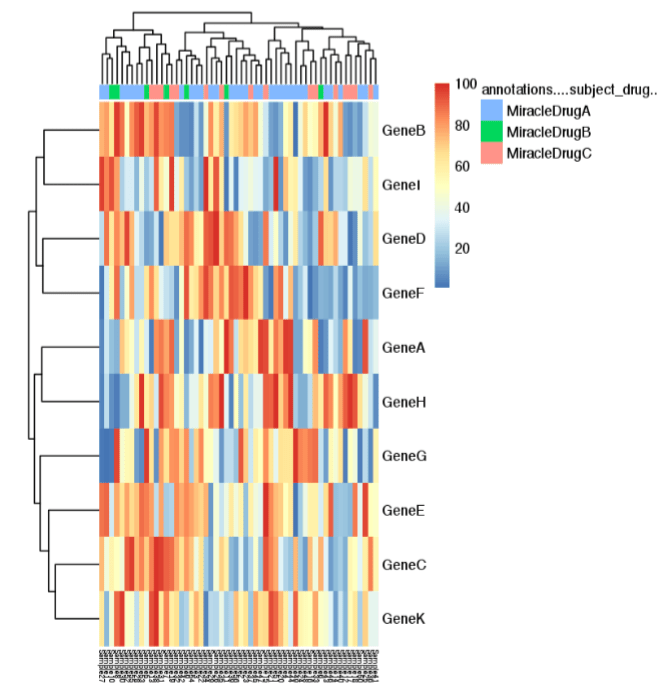It also wraps long text lines: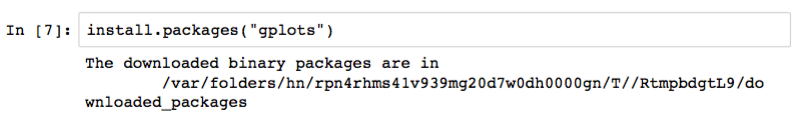and fits tables to the page width, where possible: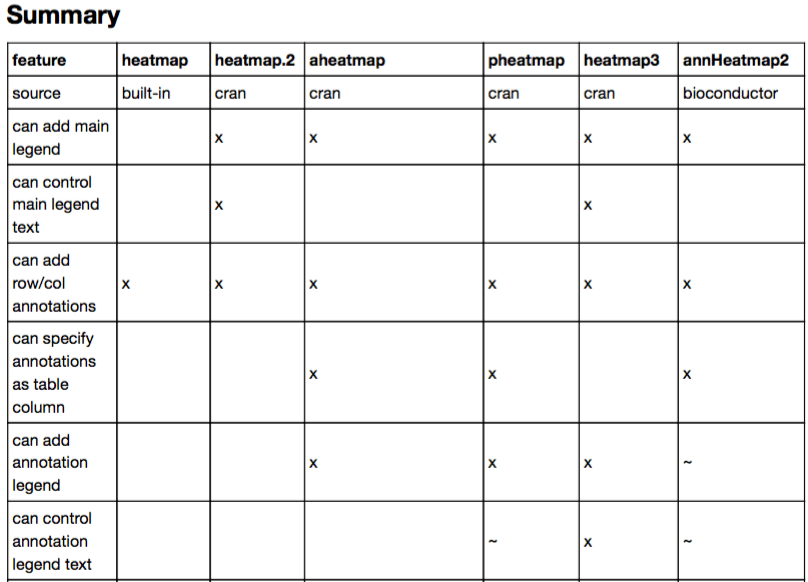You still (of course) lose the view of very wide tables, as the HTML scroll bars don’t work in PDF, but at some point you’ve got to accept that you can’t fit 10 pounds in a 5-pound sack!The "print to PDF" option in the browser also reproduces the appearance of the HTML notebook much more faithfully than Jupyter `nbconvert`‘s PDF conversion, which imposes a very LaTeX-y format (this of course makes sense, as the `nbconvert` PDF conversion goes by way of a trip through LaTeX). Finally, "Print to PDF" is also noticeably faster than `nbconvert`, although the time it takes to generate a PDF either way is unlikely to be a bottleneck!